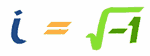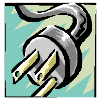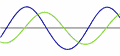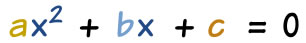# 虚数

 虚数的平方是负数。## 尝试

• 2 × 2 = 4
• (−2) × (−2) = 4（因为负负得正
• 0 × 0 = 0
• 0.1 × 0.1 = 0.01…… 但们可以想象有这样的数（称之为 i）： i × i = −1 有什么用？可以用来做什么？意思是 i 是 −1 的平方根。

…… 接受 i 的存在后，我们可以解答很多

（见怎样简化平方根

√(−x) = i√x

### 例子：解 x2 = −1

x = ± √(−1)
x = ± i

• (−i)2 = (−i)(−i) = +i2 = −1
• (+i)2 = (+i)(+i) = +i2 = −1## 虚数例子

 i 12.38i −i 3i/4 0.01i −i/2

## 虚数很有用### 频谱分析仪### 电学AC（交流电）是随时间按正弦波作周期性变化的电流。### 二次方程## 有趣属性

 i × i = −1, 然后 −1 × i = -i, 然后 −i × i = 1, 然后 1 × i = i（又回到 i）i = √−1 i2 = −1 i3 = −√−1 i4 = 1 i5 = √−1 ……

### 例子：i6 是多少？

 i6 = i4 × i2 = 1 × −1 = −1## 结论